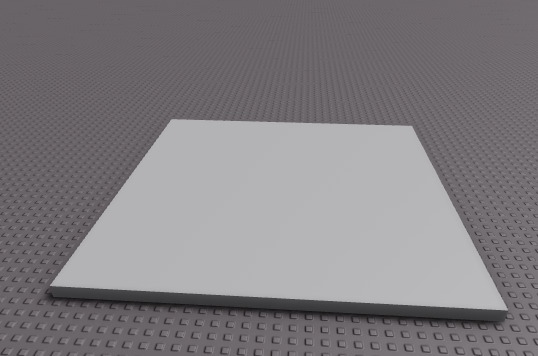# Models CFrame isn't going to it's set CFrame

1. What do you want to achieve?
I want the tree model to spawn on this part at a random position.1. What is the issue?
The tree model isn’t going anywhere near the area where it’s supposed to spawn. It just goes to the 0,0,0 position.

2. What solutions have you tried so far?
I’ve tried setting a part to its CFrame but the part actually goes to where the CFrame is but not the tree model. One problem I had with that is it didn’t go to the area where it was supposed to be.

I’ve tried setting the tree model to an already set tree model that I placed before I ran the script but that didn’t work either.

I printed the PrimaryPartCFrame of the tree model and it printed out the numbers of where it was supposed to be.

Script to make it spawn at random location

``````local tree = game.ReplicatedStorage.Tree

local cree = tree:Clone()
cree:SetPrimaryPartCFrame(CFrame.new(math.random(-56,83),5,math.random(24,51)))
``````local tree = game.ReplicatedStorage.Tree